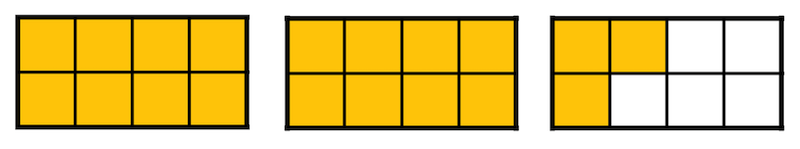# Adding Fractions with Whole Numbers Overview

A mixed number is a number that has a whole number part and a fractional part.

Adding Fractions with Whole Numbers Sample Questions

Here is a visual representation of a mixed number.This model shows two rectangles that are shaded completely, which represents whole numbers, and one rectangle that is shaded partially, which represents a fraction.

This fraction model represents the mixed number $$2\frac{3}{8}$$.

When adding a mixed number with a whole number, we first add the whole numbers, then include the fraction.

### Example:

What is the sum of $$11\frac{2}{3}$$ and $$19$$?

We will start by adding the whole numbers, which is $$11+19=30$$. Then we add the fractional part to the end.

Therefore, the sum of $$11\frac{2}{3}$$ and $$19$$ is $$30\frac{2}{3}$$.

### Here is an example of where this can be used in real life:

Monica picks two bags of peaches to buy at the Farmer’s Market. She places each bag on the scale and the first bag weighs $$5$$ lbs. and the second bag weighs $$6\frac{2}{3}$$ lbs. How many pounds of peaches does Monica buy?

When adding a whole number and a fraction we first add the whole numbers, then we include the fraction.

$$5+6=11$$, now we include $$\frac{2}{3}$$, therefore, Monica buys a total of $$11\frac{2}{3}$$ lbs. of peaches.

## Adding Fractions with Whole Numbers Sample Questions

Here are a few sample questions going over adding fractions with whole numbers.

Question #1:

Calculate the sum of $$14\frac{5}{6}$$ and $$38$$.

$$54$$
$$52\frac{5}{6}$$
$$56\frac{2}{5}$$
$$55$$

When adding fractions and whole numbers, first calculate the whole number plus the whole number, and then include the remaining fraction in the answer. For example, $$14+38=52$$, so $$52\frac{5}{6}$$ is the answer.

Question #2:

Calculate the sum of $$45$$ and $$2\frac{1}{3}$$.

$$47\frac{2}{3}$$
$$45\frac{2}{3}$$
$$46\frac{3}{5}$$
$$47\frac{1}{3}$$

Once again, when adding fractions and whole numbers, first calculate the whole number plus the whole number, and then include the remaining fraction in the answer. For example, $$45+2=47$$, so $$47\frac{1}{3}$$ is the answer.

Question #3:

Add $$4\frac{3}{2}+5$$.

$$10\frac{1}{5}$$
$$11\frac{3}{5}$$
$$9\frac{1}{5}$$
$$10\frac{1}{2}$$

The first step is to address the improper fraction within the mixed number $$4\frac{3}{2}$$. The fraction $$\frac{3}{2}$$ is the same thing as $$1\frac{1}{2}$$, so rewrite $$4\frac{3}{2}$$ as $$5\frac{1}{2}$$. Now, simply combine $$5\frac{1}{2}$$ and $$5$$ to get $$10\frac{1}{2}$$.

Question #4:

Add $$3+3\frac{5}{4}$$.

$$6\frac{1}{4}$$
$$7\frac{1}{4}$$
$$6\frac{3}{4}$$
$$7\frac{3}{4}$$

The first step is to address the improper fraction within the mixed number $$3\frac{5}{4}$$. The fraction $$\frac{5}{4}$$ is the same thing as $$1\frac{1}{4}$$, so rewrite $$3\frac{5}{4}$$ as $$4\frac{1}{4}$$. Now, simply combine $$3$$ and $$4\frac{1}{4}$$ to get $$7\frac{1}{4}$$.

Question #5:

Fill in the missing value in order to make the equation true.
$$3\frac{4}{5}+$$ ______$$=18\frac{4}{5}$$

$$13$$
$$14\frac{1}{5}$$
$$15$$
$$16\frac{1}{5}$$
In order to create a balanced equation, the mixed number $$18\frac{4}{5}$$ must be on each side. Adding $$3\frac{4}{5}+15=18\frac{4}{5}$$, so $$15$$ is the missing value.